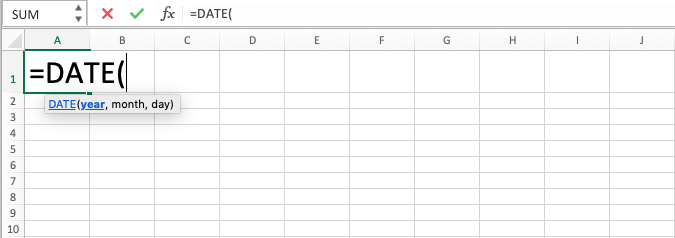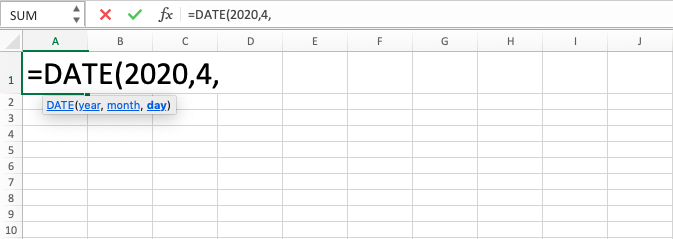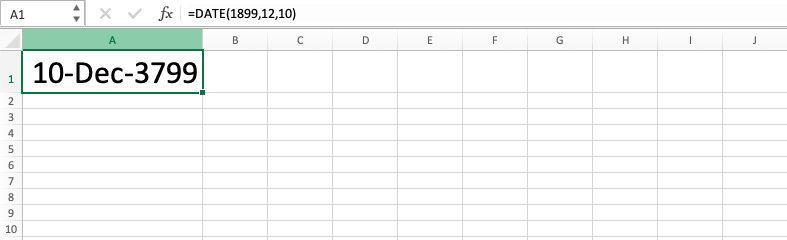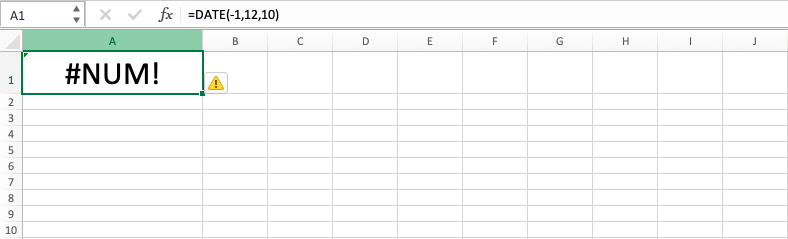DATE Formula in Excel - Compute Expert

# DATE Formula in Excel

In this tutorial, you will learn about DATE function in excel. DATE formula in excel is useful to produce unified data from the inputs of day, month, and year numeral data given by you in this formula.

## Why We Need to Learn About DATE Function in Excel?

In doing data processing related to day period in excel, sometimes we need to make this kind of data from our day, month, and year separate digits. If the digits to unite is static and the amounts are not too many, then probably it is better and faster to do it manually. But, if there are high amounts of them and their value can be changed all the time, then it will be very troublesome if we do it the standard way.

Moreover, sometimes we also need this kind of data as a criteria in formulas like SUMIF or AVERAGEIF. It is a bit difficult if we form it manually each time from the numbers of day, month, and year in each data entry if there are many data entries that need the based on criteria calculation process from the formulas like SUMIF or AVERAGEIF.

As a case example, let’s say we have our company’s daily sell and buy transaction records data on a spreadsheet with special columns for the numeral data of days, months, and years. If there is a need to make a column filled with the merging of all those numbers, then it will be very troublesome to do it manually considering the high amount of transaction record rows of a company which that is often the case in the spreadsheet.

For the usage examples in other formulas, let’s say we are in the middle of adding up the transaction data directly for a certain period of time. If there are only days, months and years digits to help us in inputting the data for per data entry evaluation in relation with the period criteria that is needed in the process, then it will be difficult if you want to do the calculation process directly even if the calculation process has been helped by using formulas such as SUMIF or COUNTIF.

In helping the process to get the unified data from the figures of day, month, and year faster and more automatically to answer needs like above, then you can use DATE formula in excel. By looking at its usefulness, DATE function in excel will be very helpful if we need date data specifically for the data processing that we are going to do.

## What is DATE Formula in Excel?

DATE formula in excel is a formula with the usage objective to create unified data from the inputs of day, month, and year figures. DATE function in excel is often used in formulas with the need for criteria based on dates kind of data or in the calculation process of this kind of data.

Here is a little bit explanation about the inputs in this formula:

=DATE(year, month, day)

Note:
• year = year number that you want to make the date from
• month = month number that you want to make the date from
• day = day number that you want to make the date from

## How to Use DATE Function in Excel?

The following will explain how to use DATE formula in excel. DATE function in excel needs inputs such as, as being described a few times previously, the figures of day, month, and year from you to be merged by it.

## Using DATE Formula in Excel

1. Type equal sign ( = ) in the cell where you want to put the result in2. Type DATE (can be with large and small letters) and open bracket sign after =3. Type year number or cell coordinate where the year number that you want to process is after open bracket sign, then type a comma sign ( , )4. Type month number or cell coordinate where the month number that you want to process is, then type a comma sign5. Type day number or cell coordinate where the day number that you want to process is6. Type close bracket sign7. Press Enter
8. The DATE function in excel process is done!## Exercise

After you have learned how to use the DATE function in excel above, you can practice your understanding by doing the exercise below!

### Questions

1. Merge all the digits in the first row into one data!
2. What is the amount of days difference between today and the day, month, and year in the second row? (Instruction: use TODAY() formula to get the data you need for today!)
3. Is the day, month, and year in the third row is more than 15 December 1999? Answer with “Yes” or “No”! (Instruction: use DATE formula in excel and IF function to compare the two!)

• The earliest year can be inputted in DATE function in excel is 1900. If you try to input the digit between 0 to 1899 for the year input, then the digit will be added with 1900 for the final result. See the picture below for the exampleIf the input in the year part is minus, then the result will be #NUM error• If you input minus numeral data for the month, then DATE formula in excel will adjust the year with the minus digit of the month that you inputIf the month input is more than 12, then DATE function in excel will adjust the year with the excess amount of 12 in your input• If you input minus numeral data for the day, then DATE formula in excel will adjust the month and year with the minus digit of the day that you inputIf the day input is more than the days’ amount in the inputted month, then DATE function in excel will adjust to the month and year in line with the excess amount of day in your input• You can change the date format by using Format Cells menu or TEXT formula. If you want to use TEXT function, then you can learn it from the other part of Compute Expert blog if you still don’t understand how to use it!

Get updated excel info from Compute Expert by registering your email. It's free!